Download Presentation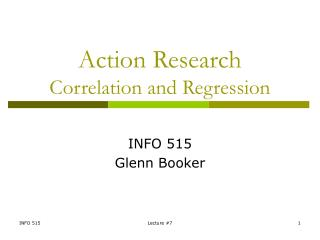Action Research Correlation and Regression

# Action Research Correlation and Regression - PowerPoint PPT Presentation

Action Research Correlation and Regression. INFO 515 Glenn Booker. Measures of Association. Measures of association are used to determine how strong the relationship is between two variables or measures, and how we can predict such a relationshipI am the owner, or an agent authorized to act on behalf of the owner, of the copyrighted work described.
Download Presentation## Action Research Correlation and Regression

An Image/Link below is provided (as is) to download presentation

Download Policy: Content on the Website is provided to you AS IS for your information and personal use and may not be sold / licensed / shared on other websites without getting consent from its author.While downloading, if for some reason you are not able to download a presentation, the publisher may have deleted the file from their server.

- - - - - - - - - - - - - - - - - - - - - - - - - - E N D - - - - - - - - - - - - - - - - - - - - - - - - - -
Presentation Transcript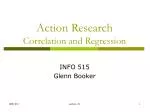### Action ResearchCorrelation and Regression

INFO 515

Glenn Booker

Lecture #7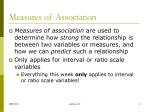Measures of Association
• Measures of association are used to determine how strong the relationship is between two variables or measures, and how we can predict such a relationship
• Only applies for interval or ratio scale variables
• Everything this week only applies to interval or ratio scale variables!

Lecture #7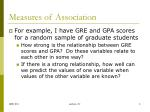Measures of Association
• For example, I have GRE and GPA scores for a random sample of graduate students
• How strong is the relationship between GRE scores and GPA? Do these variables relate to each other in some way?
• If there is a strong relationship, how well can we predict the values of one variable when values of the other variable are known?

Lecture #7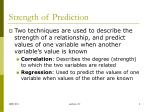Strength of Prediction
• Two techniques are used to describe the strength of a relationship, and predict values of one variable when another variable’s value is known
• Correlation: Describes the degree (strength) to which the two variables are related
• Regression: Used to predict the values of one variable when values of the other are known

Lecture #7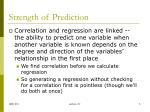Strength of Prediction
• Correlation and regression are linked -- the ability to predict one variable when another variable is known depends on the degree and direction of the variables’ relationship in the first place
• We find correlation before we calculate regression
• So generating a regression without checking for a correlation first is pointless (though we’ll do both at once)

Lecture #7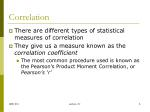Correlation
• There are different types of statistical measures of correlation
• They give us a measure known as the correlation coefficient
• The most common procedure used is known as the Pearson’s Product Moment Correlation, or Pearson’s ‘r’

Lecture #7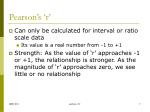Pearson’s ‘r’
• Can only be calculated for interval or ratio scale data
• Its value is a real number from -1 to +1
• Strength: As the value of ‘r’ approaches -1 or +1, the relationship is stronger. As the magnitude of ‘r’ approaches zero, we see little or no relationship

Lecture #7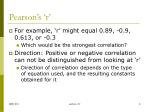Pearson’s ‘r’
• For example, ‘r’ might equal 0.89, -0.9, 0.613, or -0.3
• Which would be the strongest correlation?
• Direction: Positive or negative correlation can not be distinguished from looking at ‘r’
• Direction of correlation depends on the type of equation used, and the resulting constants obtained for it

Lecture #7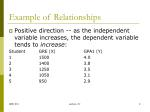Example of Relationships
• Positive direction -- as the independent variable increases, the dependent variable tends to increase:

Student GRE (X) GPA1 (Y)

1 1500 4.0

2 1400 3.8

3 1250 3.5

4 1050 3.1

5 950 2.9

Lecture #7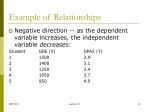Example of Relationships
• Negative direction -- as the dependent variable increases, the independent variable decreases:

Student GRE (X) GPA2 (Y)

1 1500 2.9

2 1400 3.1

3 1250 3.4

4 1050 3.7

5 950 4.0

Lecture #7Positive correlation, r = 1.0

Negative correlation, r = 1.0

Positive and Negative Correlation

Data from slide 9

Data from slide 10

Notice that high ‘r’ doesn’t tell whether the correlation is positive or negative!

Lecture #7*Important Note*
• An association value provided by a correlation analysis, such as Pearson’s ‘r’, tells us nothing about causation
• In this case, high GRE scores don’t necessarily cause high or low GPA scores, and vice versa

Lecture #7Significance of r
• We can test for the significance of r (to see whether our relationship is statistically significant) by consulting a table of critical values for r (Action Research p. 41/42)
• Table “VALUES OF THE CORRELATION COEFFICIENT FOR DIFFERENT LEVELS OF SIGNIFICANCE”
• Where df = (number of data pairs) – 2

Lecture #7Significance of r
• We test the null hypothesis that the correlation between the two variables is equal to zero (there is no relationship between them)
• Reject the null hypothesis (H0) if the absolute value of r is greater than the critical r value
• Reject H0 if |r| > rcrit
• This is similar to evaluating actual versus critical ‘t’ values

Lecture #7Significance of r Example
• So if we had 20 pairs of data
• For two-tail 95% confidence (P=.05), the critical ‘r’ value at df=20-2=18 is 0.444
• So reject the null hypothesis (hence correlation is statistically significant) if:
• r > 0.444 or r < -0.444

Lecture #7Strength of “|r|”
• Absolute value of Pearson’s ‘r’ indicates the strength of a correlation
• 1.0 to 0.9: very strong correlation
• 0.9 to 0.7: strong
• 0.7 to 0.4: moderate to substantial
• 0.4 to 0.2: moderate to low
• 0.2 to 0.0: low to negligible correlation
• Notice that a correlation can be strong, but still not be statistically significant! (especially for small data sets)

Lecture #7*Important Notes*
• The stronger the r, the smaller the standard estimate of the error, the better the prediction!
• A significant r does not necessarily mean that you have a strong correlation
• A significant r means that whatever correlation you do have is not due to random chance

Lecture #7Coefficient of Determination
• By squaring r, we can determine the amount of variance the two variables share (called “explained variance”)
• R Square is the coefficient of determination
• So, an “R Square” of 0.94 means that 94% of the variance in the Y variable is explained by the variance of the X variable

Lecture #7What is R Squared?
• The Coefficient of determination, R2, is a measure of the goodness of fit
• R2 ranges from 0 to 1
• R2 = 1 is a perfect fit (all data points fall on the estimated line or curve)
• R2 = 0 means that the variable(s) have no explanatory power

Lecture #7What is R Squared?
• Having R2 closer to 1 helps choose which regression model is best suited to a problem
• Having R2 actually equal zero is very difficult
• A sample of ten random numbers from Excel still obtained an R2 of 0.006

Lecture #7Scatter Plots
• It’s nice to use R2 to determine the strength of a relationship, but visual feedback helps verify whether the model fits the data well
• Also helps look for data fliers (outliers)
• A scatter plot (or scatter gram) allows us to compare any two interval or ratio scale variables, and see how data points are related to each other

Lecture #7Scatter Plots
• Scatter plots are two-dimensional graphs with an axis for each variable (independent variable X and dependent variable Y)
• To construct: place an * on the graph for each X and Y value from the data
• Seeing data this way can help choose the correct mathematical model for the data

Lecture #7Y(Dep.)

X=2

Data point (2, 3)

*

Y=3

(0, 0)

X(Indep.)

Scatter Plots

Lecture #7Models
• Allow us to focus on select elements of the problem at hand, and ignore irrelevant ones
• May show how parts of the problem relate to each other
• May be expressed as equations, mappings, or diagrams
• May be chosen or derived before or after measurement (theory vs. empirical)

Lecture #7Modeling
• Often we look for a linear relationship – one described by fitting a straight line as well to the data as possible
• More generally, any equation could be used as the basis for regression modeling, or describing the relationship between two variables
• You could have Y = a*X**2 + b*ln(X) + c*sin(d*X-e)

Lecture #7Y = m*X + b or Y = b0 + b1*X

Y(Dep.)

m = slope

1 unit of X

b = Y axis intercept

X(Indep.)

Linear Model

Lecture #7Linear Model
• Pearson’s ‘r’ for linear regression is calculated per (Action Research p. 29/30)
• Define: N = number of data pairs SX = Sum of all X values SX2 = Sum of all (X values squared) SY = Sum of all Y values SY2 = Sum of all (Y values squared) SXY = Sum of all (X values times Y values)
• Pearson’s r = [N*(SXY) – (SX)*(SY)] / sqrt[(N*(SX2) – (SX)^2)*(N*(SY2) – (SY)^2)]

Lecture #7Linear Model
• For the linear model, you could find the slope ‘m’ and Y-intercept ‘b’ from
• m = (r) * (standard deviation of Y) / (standard deviation of X)
• b = (mean of Y) – (m)*(mean of X)
• But it’s a lot easier to use SPSS’ slope=b1 and Y intercept = b0

Lecture #7Regression Analysis
• Allows us to predict the likely value of one variable from knowledge of another variable
• The two variables should be fairly highly correlated (close to a straight line)
• The regression equation is a mathematical expression of the relationship between 2 variables on, for example, a straight line

Lecture #7Regression Equation
• Y = mX + b
• In this linear equation, you predict Y values (the dependent variable) from known values of X (the independent variable); this is called the regression of Y on X
• The regression equation is fundamentally an equation for plotting a straight line, so the stronger our correlation -- the closer our variables will fall to a straight line, and the better our prediction will be

Lecture #7Linear Regression

y

^

y

y

^

y = a + b*x

^

y = y + e

x

Choose “best” line by minimizing the sum of the squares of the vertical distances between the data points and the regression line

Lecture #7Standard Error of the Estimate
• Is the standard deviation of data around the regression line
• Tells how much the actual values of Y deviate from the predicted values of Y

Lecture #7Standard Error of the Estimate
• After you calculate the standard error of the estimate, you add and subtract the value from your predicted values of Y to get a % area around the regression line within which you would expect repeated actual values to occur or cluster if you took many samples (sort of like a sampling distribution for the mean….)

Lecture #7Standard Error of Estimate
• The Standard Error of Estimate for Y predicted by X issy/x =sqrt[sum of(Y–predicted Y)2 /(N–2)]where ‘Y’ is each actual Y value‘predicted Y’ is the Y value predicted by the linear regression‘N’ is the number of data pairs
• For example on (Action Research p. 33/34), Sy/x = sqrt(2.641/(10-2)) = 0.574

Lecture #7Standard Error of the Estimate
• So, if the standard error of the estimate is equal to 0.574, and if you have a predicted Y value of 4.560, then 68% of your actual values, with repeated sampling, would fall between 3.986 and 5.134 (predicted Y +/- 1 std error)
• The smaller the standard error, the closer your actual values are to the regression line, and the more confident you can be in your prediction

Lecture #7SPSS Regression Equations
• Instead of constants called ‘m’ and ‘b’, ‘b0’ and ‘b1’ are used for most equations
• The meaning of ‘b0’ and ‘b1’ varies, depending on the type of equation which is being modeled
• Can repress the use of ‘b0’ by unchecking “Include constant in equation”

Lecture #7SPSS Regression Models
• Linear modelY = b0 + b1*X
• Logarithmic modelY = b0 + b1*ln(X) where ‘ln’ = natural log
• Inverse model Y = b0 + b1/XSimilar to the form X*Y = constant, which is a hyperbola

Lecture #7SPSS Regression Models
• Power modelY = b0*(X**b1)
• Compound model Y = b0*(b1**X)
• A variant of this is the Logistic model, which requires a constant input ‘u’ which is larger than Y for any actual data pointY = 1/[ 1/u + b0*(b1**X) ]

Where “**” indicates “to the power of”

Lecture #7SPSS Regression Models

“exp” means “e to the power of”;e = 2.7182818…

• Exponential model Y = b0*exp(b1*X)
• Other exponential functions
• S modelY = exp(b0 + b1/X)
• Growth model (is almost identical to the exponential model)Y = exp(b0 + b1*X)

Lecture #7SPSS Regression Models
• Polynomials beyond the Linear model (linear is a first order polynomial):
• Quadratic (second order)Y = b0 + b1*X + b2*X**2
• Cubic (third order)Y = b0 + b1*X + b2*X**2 + b3*X**3These are the only equations which use constants b2 & b3
• Higher order polynomials require the Regression module of SPSS, which can do regression using any equation you enter

Lecture #7Y = whattheflock?
• To help picture these equations
• Make an X variable over some typical range (0 to 10 in a small increment, maybe 0.01)
• Define a Y variable
• Calculate the Y variable using Transform > Compute… and whatever equation you want to see
• Pick values for b0 and b1 that aren’t 0, 1, or 2
• Have SPSS plot the results of a regression of Y vs X for that type of equation

Lecture #7How Apply This?
• Given a set of data containing two variables of interest, generate a scatter plot to get some idea of what the data looks like
• Choose which types of models are most likely to be useful
• For only linear models, use Analyze / Regression / Linear...

Lecture #7How Apply This?
• Select the Independent (X) and Dependent (Y) variables
• Rules may be applied to limit the scope of the analysis, e.g. gender=1
• Dozens of other characteristics may also be obtained, which are beyond our scope here

Lecture #7How Apply This?
• Then check for the R Square value in the Model Summary
• Check the Coefficients to make sure they are all significant (e.g. Sig. < 0.050)
• If so, use the ‘b0’ and ‘b1’ coefficients from under the ‘B’ column (see Statistics for Software Process Improvement handout), plus or minus the standard errors “SE B”

Lecture #7Regression Example
• For example, go back to the “GSS91 political.sav” data set
• Generate a linear regression (Analyze > Regression > Linear) for ‘age’ as the Independent variable, and ‘partyid’ as the Dependent variable
• Notice that R2 and the ANOVA summary are given, with F and its significance

Lecture #7Regression Example
• The R Square of 0.006 means there is a very slight correlation (little strength)
• But the ANOVA Significance well under 0.050 confirms there is a statistically significant relationship here - it’s just a really weak one

Lecture #7Output from Analyze > Regression > Linear

Output from Analyze > Regression > Curve Estimation

Regression Example

Lecture #7Regression Example
• The heart of the regression analysis is in the Coefficients section
• We could look up ‘t’ on a critical values table, but it’s easier to:
• See if all values of Sig are < 0.050 - if they are, reject the null hypothesis, meaning there is a significant relationship
• If so, use the values under B for b0 and b1
• If any coefficient has Sig > 0.050, don’t use that regression (coeff might be zero)

Lecture #7Regression Example
• The answer for “what is the effect of age on political view?” is that there is a very weak but statistically significant linear relationship, with a reduction of 0.009 (b1) political view categories per year
• From the Variable View of the data, since low values are liberal and large values conservative, this means that people tend to get slightly more liberal as they get older

Lecture #7Curve Estimation Example
• For the other regression options, choose Analyze / Regression / Curve Estimation…
• Define the Dependents (variable) and the Independent variable - note that multiple Dependents may be selected
• Check which math models you want used
• Display the ANOVA table for reference

Lecture #7Curve Estimation Example
• SPSS Tip: up to three regression models can be plotted at once, so don’t select more than that if you want a scatter plot to go with the data and the regressions
• For the same example just used, get a summary for the linear and quadratic models (Analyze > Regression > Curve Estimation)
• Find “R Square” for each model
• Generally pick the model with largest R Square
• Already saw Linear output, now see Quadratic

Lecture #7Curve Estimation Example
• For the quadratic regression, R Square is slightly higher, and the ANOVA is still significant

Lecture #7Curve Estimation Example
• The Quadratic coefficients are all significant at the 0.050 level

Interpret as partyid = (4.191 +/- 0.412) + (-0.048 +/- 0.018)*age + (0.0003918+/- 0.0001754)*age**2Edit the data table, then double click on the cells to get the values of b2 and its std error.

Lecture #7Curve Estimation Example
• The data set will be plotted as the Observed points, with the regression models shown for comparison
• Look to see which model most closely matches the data
• Look for regions of data which do or don’t match the model well (if any)

Lecture #7Curve Estimation Procedure
• See which models are significant (throw out the rest!)
• Compare the R Square values to see which provides the best fit
• Use the graph to verify visually that the correct model was chosen
• Use the model equation’s ‘B’ values and their standard errors to describe and predict the data’s behavior

Lecture #7